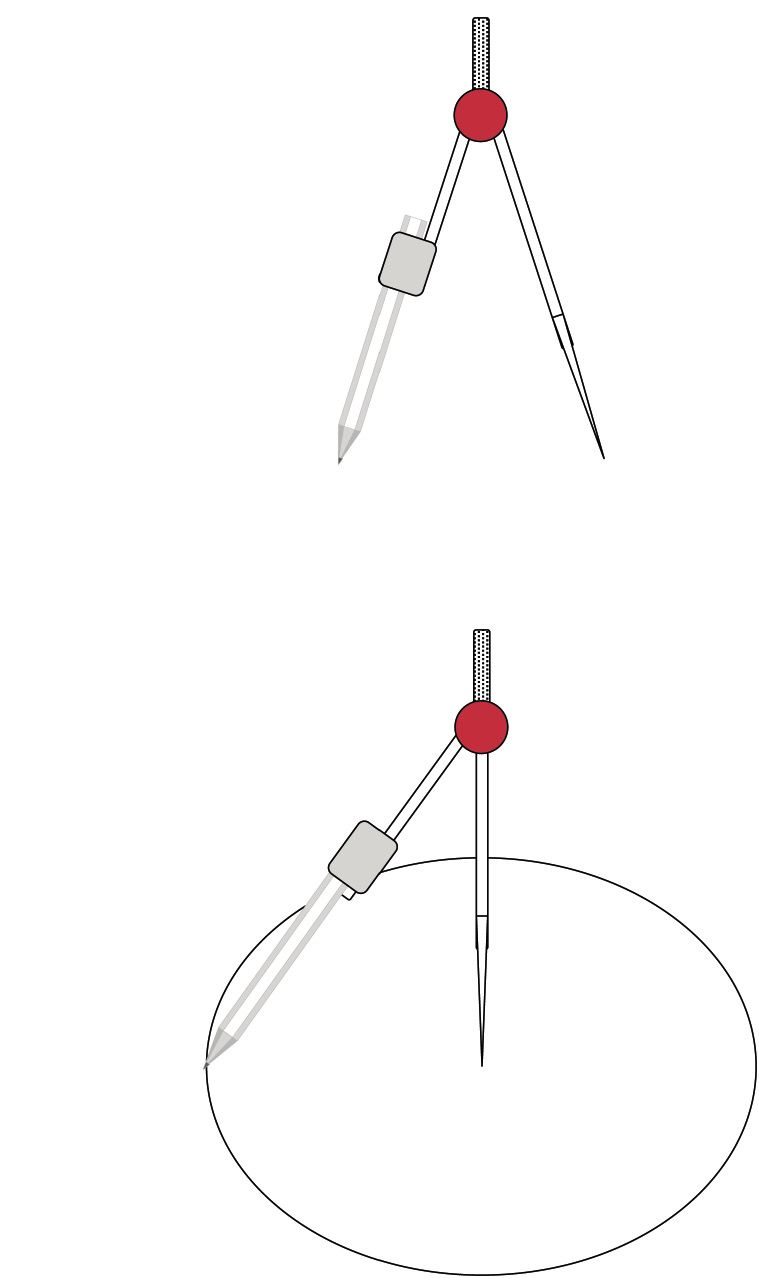perpendicular lines.
• What does it mean if we say 'two lines are perpendicular'?
• Use your protractor to measure the angles between the following pairs of lines. Then state whether they are perpendicular or not.
1.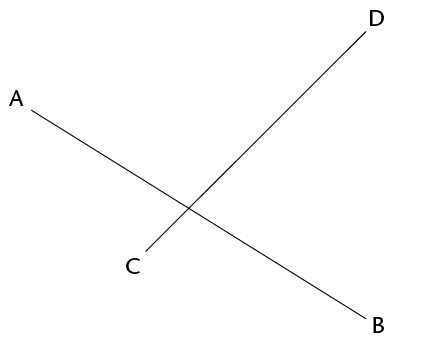2.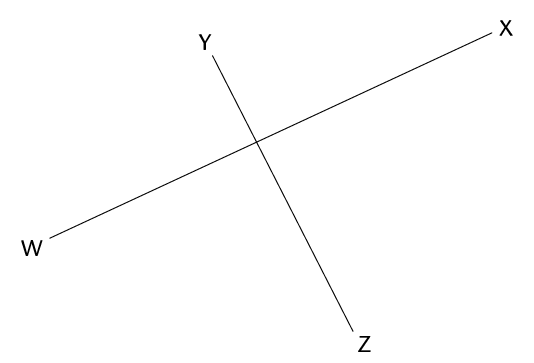• Do the following in your exercise book:
1. Use a compass to draw two overlapping circles of different sizes.
2. Draw a line through the points where the circles intersect (overlap).
3. Draw a line to join the centres of the circles.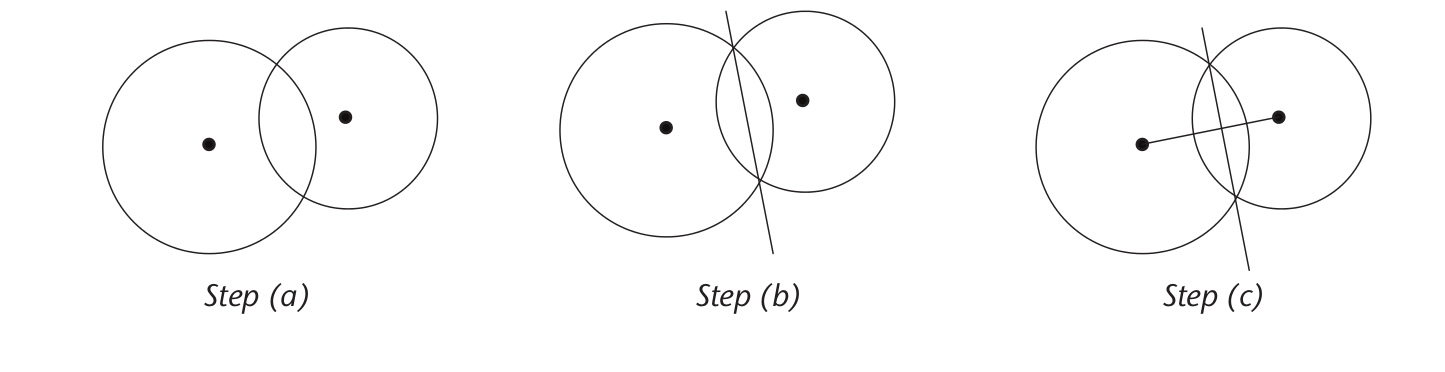4. Use your protractor to measure the angles between the intersecting lines.
5. What can you say about the intersecting lines?
• Repeat questions 1(a) to (e) with circles that are the same size.
• What conclusion can you make about a line drawn between the intersection points of two overlapping circles and a line through their centres?
• You are given line segment MN with point P at a distance from it. You must construct a line that is perpendicular to MN, so that the perpendicular passes through point P.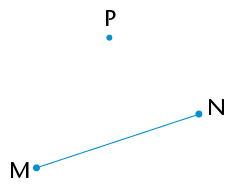Step 1

Use your compass to draw a circle whose centre is the one end point of the line segment (N) and passes through the point (P).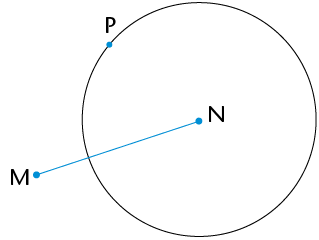Step 2

Repeat step 1, but make the centre of your circle the other end point of the line segment (M).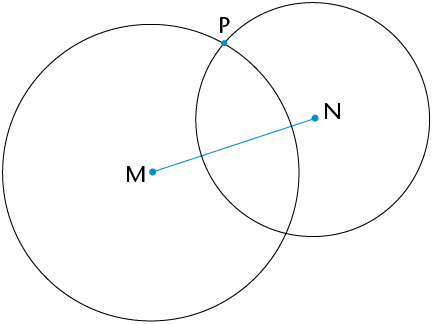Step 3

Join the points where the circles intersect: PQ $\perp$ MN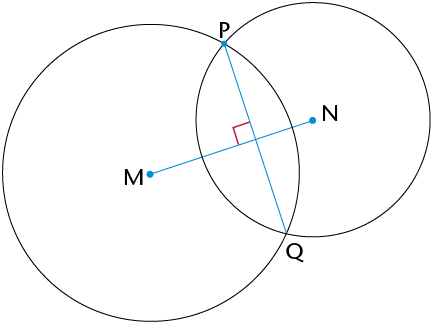You are given line segment XY with point Z on it. You must construct a perpendicular line passing through Z.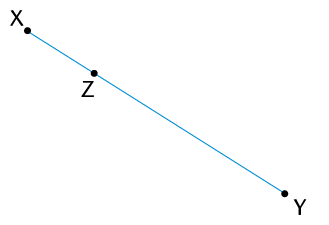Step 1

Use your compass to draw a circle whose centre is Z. Make its radius smaller than ZX. Note the two points where the circle intersects XY.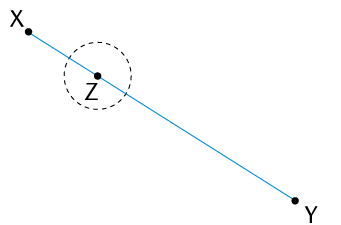Step 2

Set your compass wider than it was for the circle with centre Z. Draw two circles of the same size whose centres are at the two pointswhere the first (black) circle intersects XY. The two circles (green) will overlap.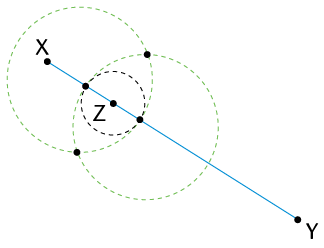Step 3

Join the intersection points of the two overlapping circles. Mark these points C and D: CD $\perp$ XY and passes through point Z.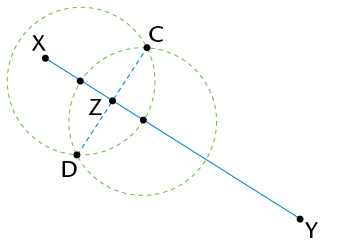•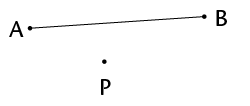•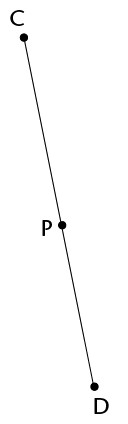• bisect an angle. Do the steps yourself in your exercise book.

The word bisect means 'to cut in half'.

You are given $\text{A}\hat{\text{B}}\text{C}$. You must bisect the angle.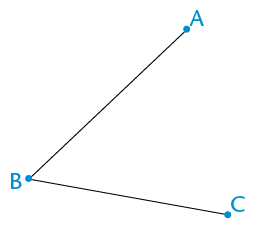Step 1

Draw a circle with centre B to mark off an equal length on both arms of the angle. Label the points of intersection D and E: DB = BE.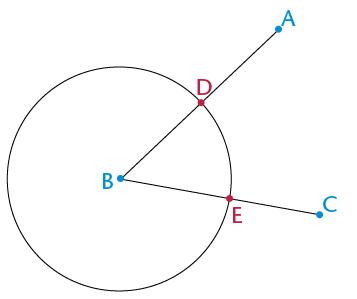Step 2

Draw two equal circles with centres at D and at E. Make sure the circles overlap.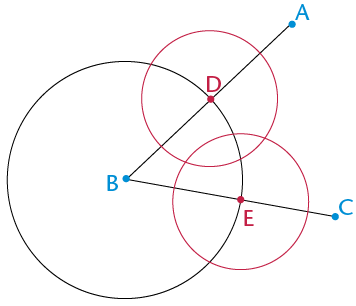Step 3

Draw a line from B through the points where the two equal circles intersect. This line will bisect the angle.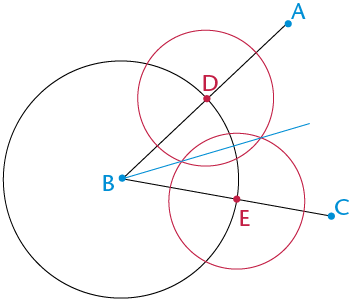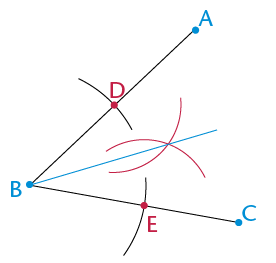Can you explain why the method above works to bisect an angle?

Can you also see that we need not draw full circles, but can merely use parts of circles (arcs) to do the above construction?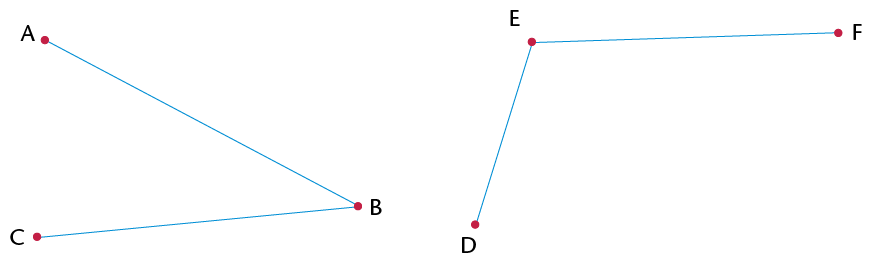special angles. You must be able to construct these angles without using a protractor.

Hint: Extend the line to the left of X.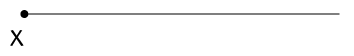• What do you know about the sides and angles in an equilateral triangle?
• In your exercise book, draw two circles with the following properties:
• The circles are the same size.
• Each circle passes through the other circle's centre.
• The centres of the circles are labelled A and B.
• The points of intersection of the circles are labelled D and E.

An example is shown here:• Draw in the following line segments: AB, AD and DB.
• What can you say about the lengths of AB, AD and DB?
• What kind of triangle is ABD?
• Therefore, what do you know about $\hat{A}, \hat{B} \text{ and } \hat{D}$?
• Use your knowledge of bisecting angles to create an angle of 30$^\circ$ on the construction you made in question 2.
• Use what you have learnt above to construct an angle of 60$^\circ$ at point P on the following line segment:
•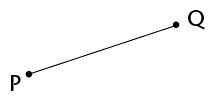• Complete the table below. The first one has been done for you:
 Angle Multiples below 360$^\circ$ Angle Multiples below 360$^\circ$ 30$^\circ$ 30$^\circ$; 60$^\circ$; 90$^\circ$; 120$^\circ$; 150$^\circ$; 180$^\circ$; 210$^\circ$; 240$^\circ$; 270$^\circ$; 300$^\circ$; 330$^\circ$ 45$^\circ$ 60$^\circ$ 90$^\circ$
• In your exercise book, construct the following angles without using a protractor. You will need to do more than one construction to create each angle.
1. 120$^\circ$
2. 135$^\circ$
3. 270$^\circ$
4. 240$^\circ$
5. 150$^\circ$
• angle bisector is a line that cuts an angle in half.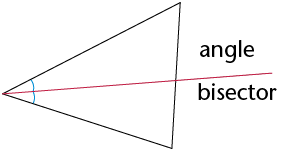1. Bisect each of the angles of the acute triangle below.
2. Extend each of the bisectors to the opposite side of the triangle.
3. What do you notice?
• Do the same with the obtuse triangle. What do you notice?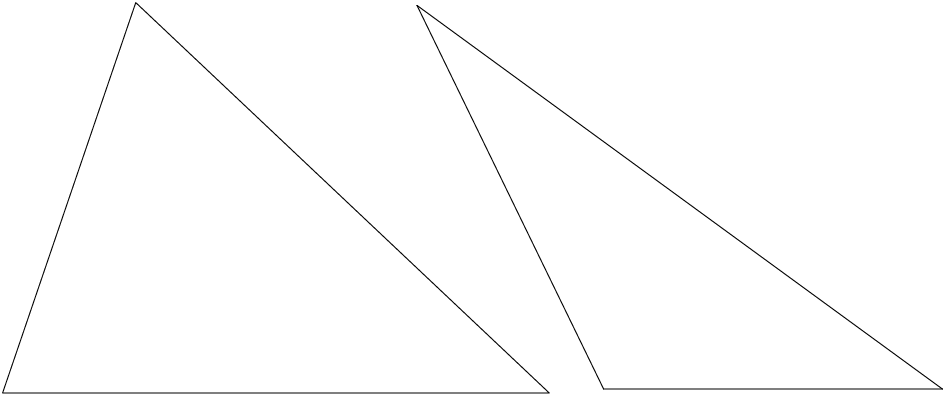• Compare your triangles with those of two classmates. You should have the same results.
• You should have found that the three angle bisectors of a triangle intersect at one point. This point is the same distance away from each side of the triangle.

An interior angle is an angle that lies between two sides of a triangle. It is inside the triangle. A triangle has three interior angles.

An exterior angle is an angle between a side of a triangle and another side that is extended. It is outside the triangle.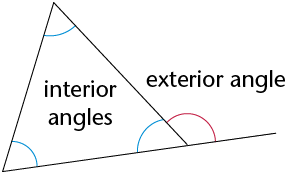interior opposite angles, as described in the following table.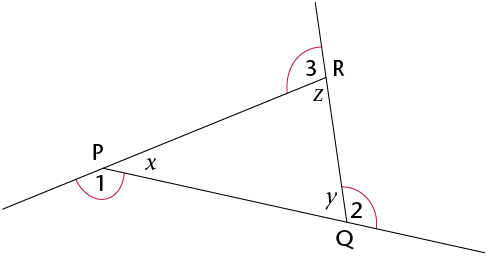Exterior angle

Interior opposite angles

1

x

z and y

2

y

x and z

3

z

x and y

• Name each exterior angle and its two interior opposite angles below.
1.2.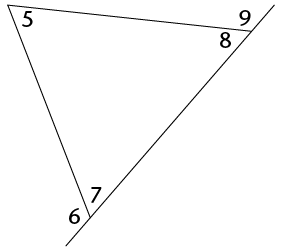3.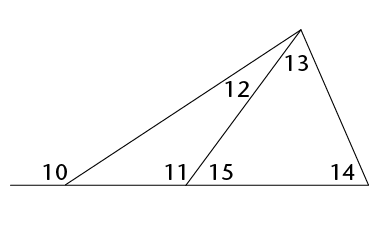Ext. $\angle$ Int. opp. $\angle$s
• $\triangle$ABC below has each side extended in both directions to create six exterior angles.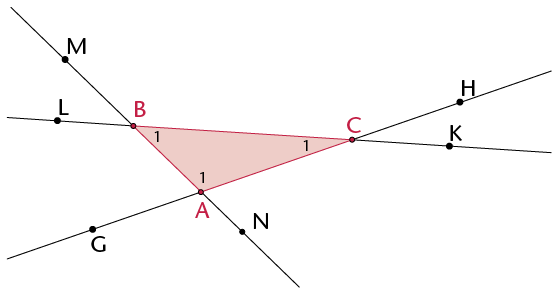1. Write down the names of the interior angles of the triangle.
2. Since a triangle has three sides that can be extended in both directions, there are two exterior angles at each vertex. Write down the names of all the exterior angles of the triangle.
3. Explain why $\text{M}\hat{\text{B}}\text{L}$ is not an exterior angle of $\triangle$ABC.

4. Write down two other angles that are neither interior nor exterior.
• Consider $\triangle$LMN. Write down the name of the exterior angle.
• Use a protractor to measure the interior angles and the exterior angle. Write the measurements on the drawing.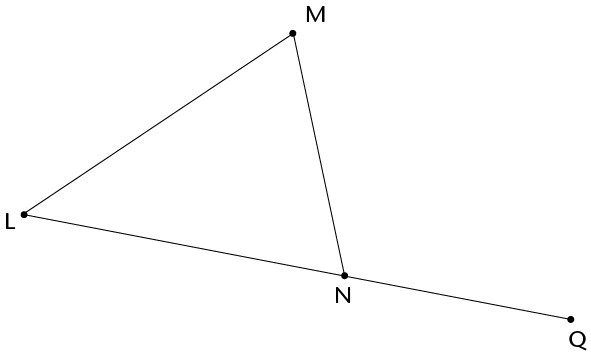• Use your findings in question 2 to complete this sum:

$\text{L}\hat{\text{M}}\text{N} + \text{M}\hat{\text{L}}\text{N} =$

• What is the relationship between the exterior angle of a triangle and the sum of the interior opposite angles?

The exterior angle of a triangle is equal to the sum of the interior opposite angles.

• Work out the sizes of angles a to f below, without using a protractor. Give reasons for the statements you make as you work out the answers.
1.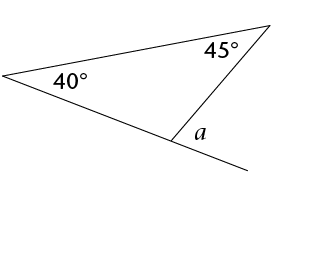2.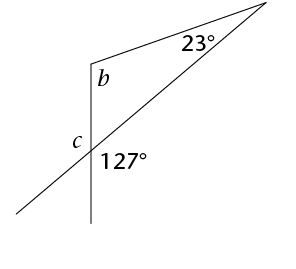3.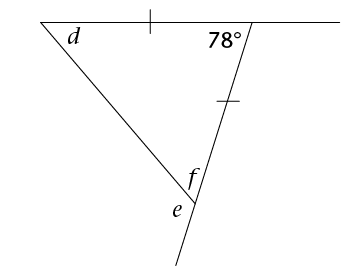• congruent if they have exactly the same shape and size: they are able to fit exactly on top of each other. This means that all three corresponding sides and three corresponding angles are equal, as shown in the following two pairs.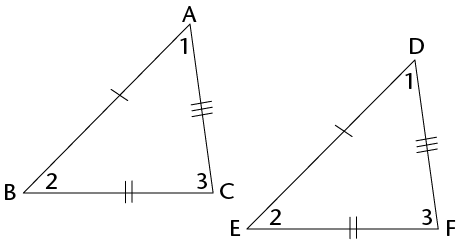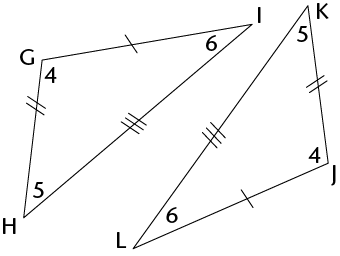• Use a ruler, compass and protractor to construct the following triangles. Each time minimum measurements are given.
1. Given three sides: side, side, side (SSS):$\triangle$DEF with DE = 7 cm, DF = 6 cm and EF = 5 cm.
2. Given three angles: angle, angle, angle (AAA): $\triangle$ABC with $\hat{\text{A}} = 80^\circ, \hat{\text{B}} = 60^\circ, \hat{\text{C}} = 40^\circ$
3. Given one side and two angles: side, angle, angle (SAA): $\triangle$GHI with $\text{GH} = 8 \text{ cm}$, $\hat{\text{G}} = 60^\circ, \hat{\text{H}} = 30^\circ$
4. Given two sides and an included angle: side, angle, side (SAS): $\triangle$JKL with JK =9 cm, $\hat{\text{K}}=$ 130$^\circ$ and KL = 7 cm.
5. Given two sides and an angle that is not included: side, side, angle (SSA):$\triangle$MNP with MN = 10 cm, $\hat{\text{M}}=$ = 50$^\circ$ and PN = 8 cm.
6. Given a right angle, the hypotenuse and a side (RHS):$\triangle$TRS with TR $\perp$ RS, RS = 7 cm and TS = 8 cm.
7. Triangle UVW with UV = 6 cm and VW = 4 cm.
• Compare your triangles with those of three classmates. Which of your triangles are congruent to theirs? Which are not congruent?
• Go back to $\triangle$MNP (1e). Did you find that you can draw two different triangles that both meet the given measurements? One of the triangles will be obtuse and the other acute. Follow the construction steps below to see why this is so.
 Step 1 Construct MN = 10 cm and the 50$^\circ$ angle at M, even though you do not know the length of unknown side (MP).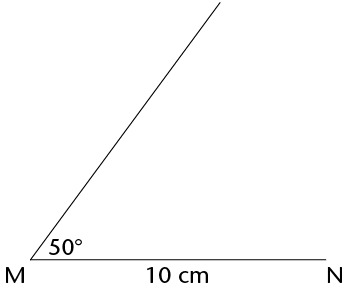Step 2 $\hat{N}$ is unknown, but NP = 8 cm. So construct an arc 8 cm from N. Every point on the arc is 8 cm from N.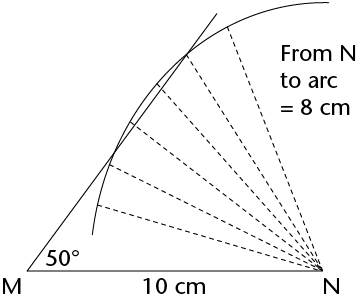Step 3 Point P must be 8 cm from N and fall on the unknown side of the triangle. The arc intersects the third side at two points, so P can be at either point. So two triangles are possible, each meeting the conditions given, i.e. MN = 10 cm, NP = 8 cm and $\hat{M}$ = 50$^\circ$.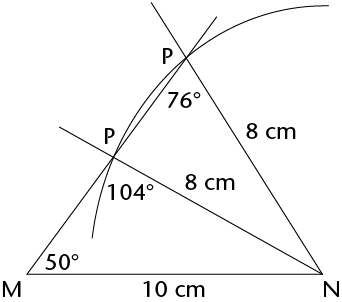• 4. Complete the table. Write down whether we can construct a congruent triangle when the following conditions are given.
 Conditions Congruent? 3 sides (SSS) 2 sides (SS) 3 angles (AAA) 2 angles and a side (AAS) 2 sides and an angle not between the sides (SSA) 2 sides and an angle between the sides (SAS) Right-angled with the hypotenuse and a side (RHS)
• diagonal is a straight line inside a figure that joins two vertices of the figure, where the vertices are not next to each other.
• Look at the quadrilaterals below. The two diagonals of the square have been drawn in: AC and BD.
• Draw in the diagonals of the other quadrilaterals below.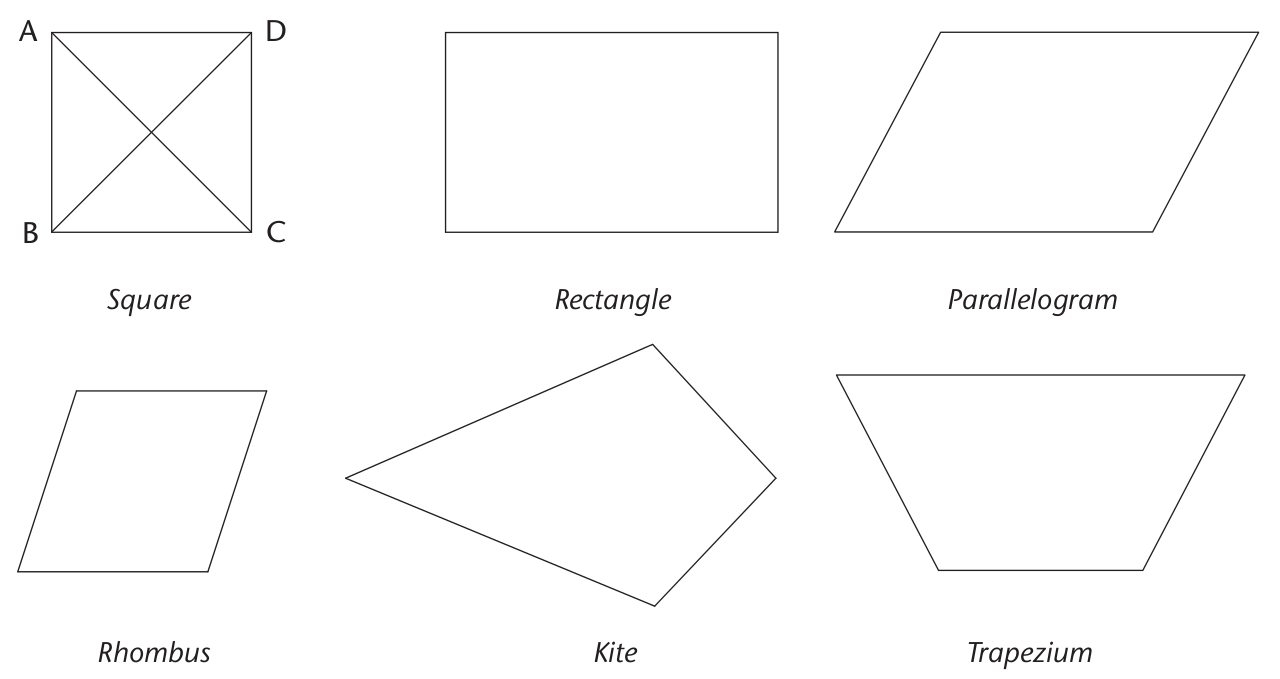• How many sides does a quadrilateral have?
• How many angles does a quadrilateral have?
• How many diagonals does a quadrilateral have?
• Construct a rhombus inside the circles by joining the centre of each circle with the intersection points of the circles. Join AB.
• Construct the perpendicular bisector of AB.(Go back to Section 10.1 if you need help.)What do you find?
• A perpendicular bisector is a line that cuts another line in half at a right angle (90$^\circ$).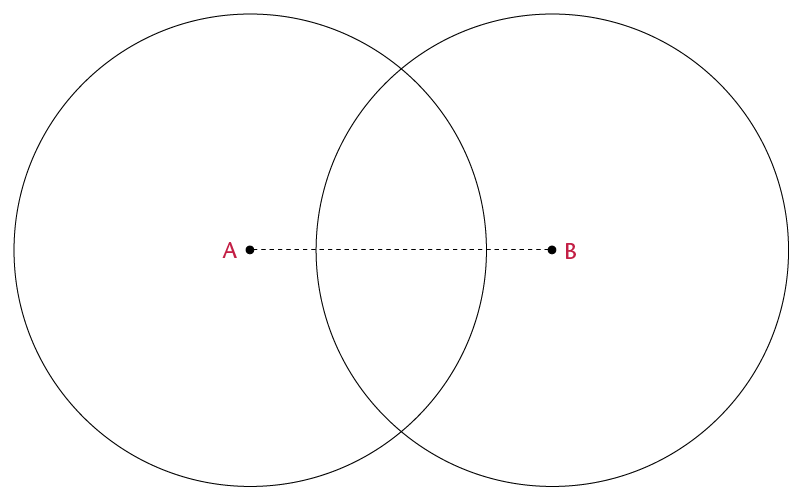• Do the diagonals bisect each other?
• Complete the sentence: The diagonals of a rhombus will always
• Construct a kite by joining the centre points of the circles to the intersection points of the circles.
• Draw in the diagonals of the kite.
• Mark all lines that are the same length.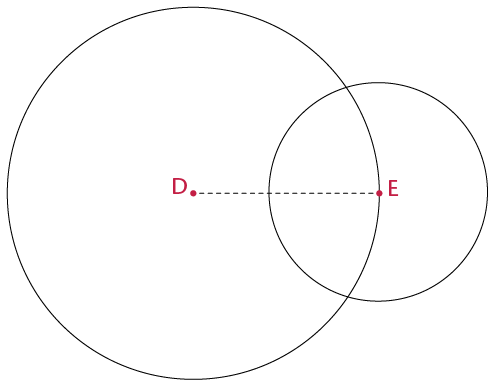• Are the diagonals of the kite perpendicular?
• Do the diagonals of the kite bisect each other?
• What is the difference between the diagonals of a rhombus and those of a kite?
• Use the grid to draw a parallelogram, rectangle and square.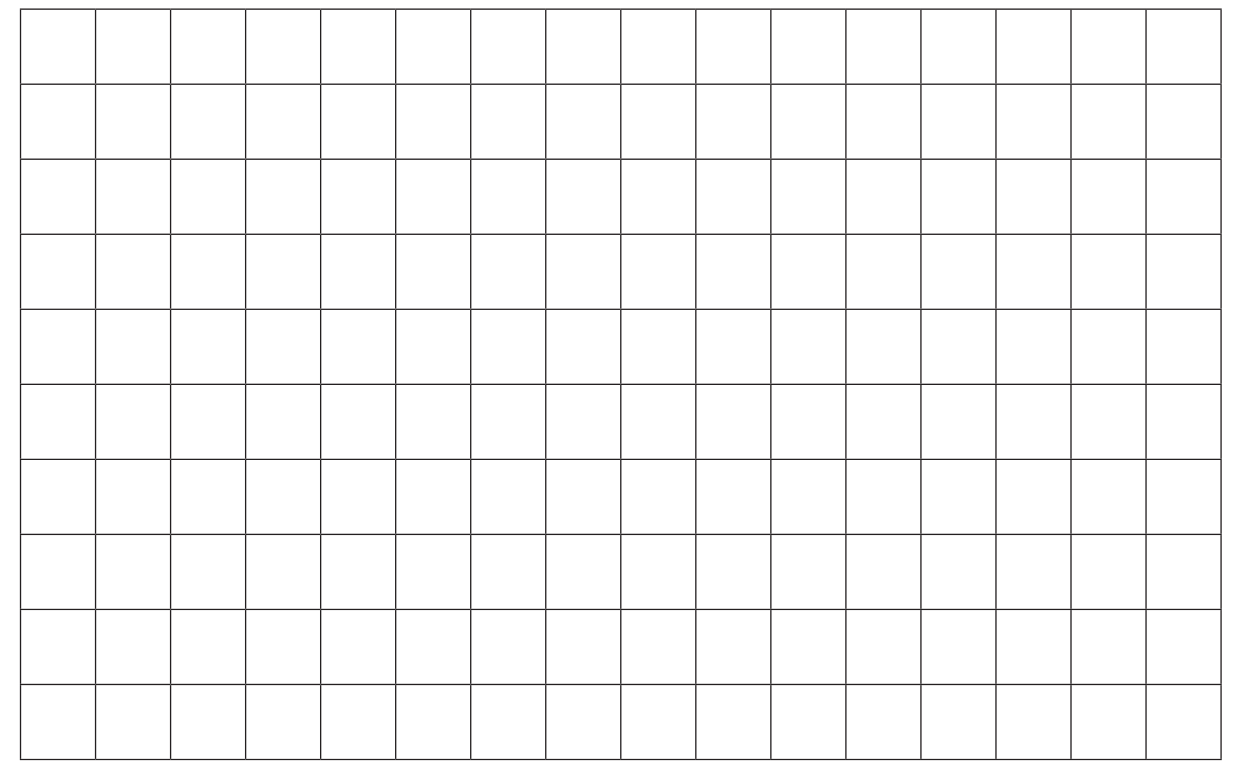• Draw in the diagonals of the quadrilaterals above.
• Indicate on each shape all the lengths in the diagonals that are equal. (Use a ruler.)
• Use the information you have found to complete the table below. Fill in 'yes' or 'no'.
 Quadrilateral Diagonals equal Diagonals bisect Diagonals meet at 90$^\circ$ Parallelogram Rectangle Square
• We can divide a quadrilateral into two triangles by drawing in one diagonal.
1. Draw in diagonals to divide each of the other polygons below into as few triangles as possible.
2. Write down the number of triangles in each polygon.
•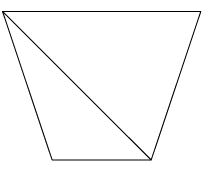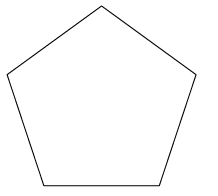Pentagon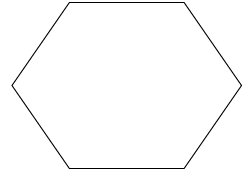Hexagon

No. of $\triangle$s

2

Sum of $\angle$s

2 \times 180$^\circ$ = 360$^\circ$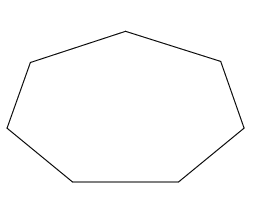Heptagon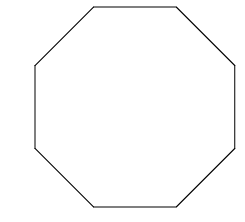Octagon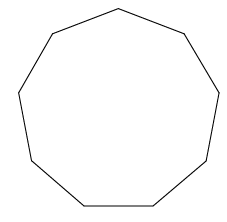Nonagon

No. of $\triangle$s

Sum of $\angle$s

• The sum of the angles of one triangle is 180$^\circ$. A quadrilateral is made up of two triangles, so the sum of the angles in a quadrilateral is $2 \times 180^\circ = 360^\circ$. Work out the sum of the interior angles of each of the other polygons above.
1. Match the words in the column on the right with the definitions on the left. Write the letter of the definition next to the matching word.
 (a) A quadrilateral that has diagonals that are perpendicular and they bisect each other Kite (b) A quadrilateral that has diagonals that are perpendicular to each other, and only one diagonal bisects the other Congruent (c) A quadrilateral that has equal diagonals that bisect each other Exterior angle (d) Figures that have exactly the same size and shape Rhombus (e) Divides into two equal parts Perpendicular (f) An angle that is formed outside a closed shape: it is between the side of the shape and a side that has been extended Bisect (g) Lines that intersect at 90 degrees Special angles (h) 90$^\circ$, 45$^\circ$, 30$^\circ$, 60$^\circ$ Rectangle
2. Complete the sentence: The exterior angle in a triangle is equal to
1. Construct $\triangle$PQR below with angles of 30$^\circ$ and 60$^\circ$. The side between the angles must be 8 cm. You may use only a ruler and a compass.
2. Will all triangles with the same measurements above be congruent to $\triangle$PQR? Explain your answer.